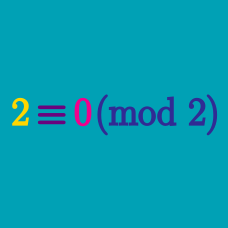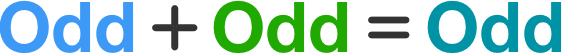Number Theory

# Modular Arithmetic - Parity, Odd/EvenTrue or False?

The sum of two odd numbers is odd.

True or False?

The sum of the squares of any three odd numbers is odd.

True or False?

The sum of the squares of three consecutive integers is always odd.

Determine the parity of

$\large 101010_3,$

that is, 101010 in base 3.

Note: In the context of number theory, the parity of a number is whether or not it is even or odd. This is different from the context of computer science, in which a parity bit counts the number of 1s in a binary code.

True or False:
The sum of an odd number and an even number is odd.

×